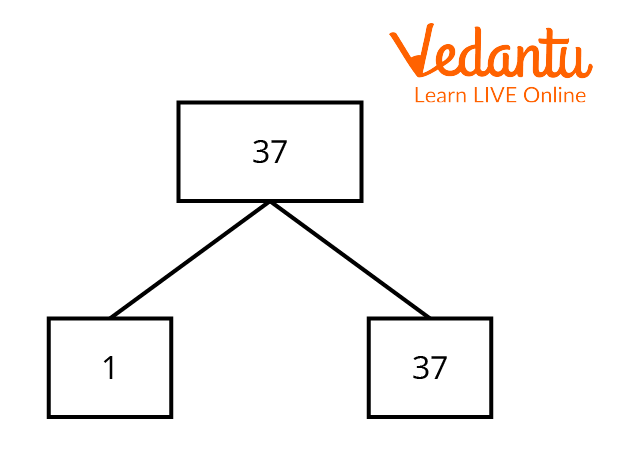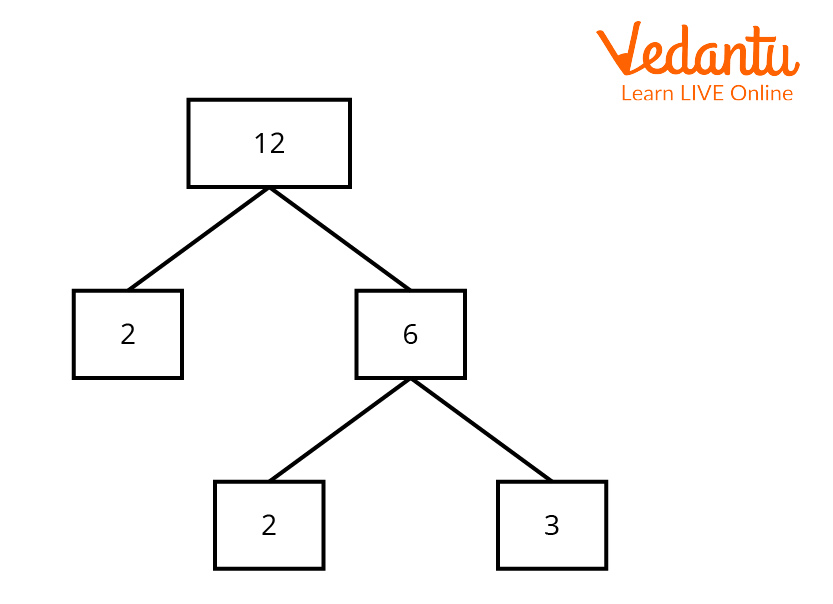Courses
Courses for Kids
Free study material
Free LIVE classes
More

# Finding the Factors for 37LIVE
Join Vedantu’s FREE Mastercalss

## Factors of 37: Preface

In this article, we will learn about the factors of 37, how to get the factors of the number 37, and the prime factors of 37, along with examples. A factor of 37 divides it evenly. These factors cannot have a fractional or decimal value. As a result, the factors are 1 and 37. When two integers are multiplied together, a factor pair of 37 is the number that provides the result of 37.

## Factors of a Number: A Brief Explanation

When the original number is divided by a factor, the result is an even number and the remainder is zero. Every number greater than one has a minimum of two factors. The two factors are known as factor products. The product of every factor pair corresponds to the number. The first factor pair is always one and counts itself.

Examples:

• The factors of 39 are 1, 3, 13, and 39

• The factors of 69 are 1, 3, 23, and 69

## What is the Factor of 37?

Factors of 37 are the numbers that divide 37 exactly with no remainder. When you multiply two whole numbers together and obtain 37 as a result, you may claim that both numbers are factors of 37. Hence, all the factors of 37 are 1 and 37.

## How to Calculate Factors for 37?

The factors of 37 are the numbers that divide 37 exactly with no remainder. 37 is an odd number, so it cannot be divided by any even integer. Determine the pairs of numbers whose product gives 37. We are aware that the exact divisors of the number 37 are its factors.

Here are all the factors for 37:

$37\div 1=37$

1 is a factor of 37.

$37\div 37=1$

37 is a factor of 37.

Thus, all the factors of 37 are 1 and 37.

## 37 is a Prime Number?

Prime factorization of 37 refers to the method of finding the prime factors of 37. We know 37 is a prime number, so we can't factor it any further, because prime numbers have no factors other than 1 and the number itself. As a result, there are no other prime factors than 37. Hence, the prime factor of 37 is $1\times 37$, where 37 is the prime number. A factor tree of 37 is given below:Factor Tree of 37

## Pair Factors of37

To get the pair factors of 37, multiply the two integers in a pair so that the result is 37. Pair factors may be positive or negative, but they cannot be a fraction or a decimal.

### Positive Factors of 37

Factor Pair of 37

$1\times 37$

$(1,37)$

Thus, the positive pair factor is $(1,37)$.

Negative pair factors are also possible since the product of two negative numbers yields a positive number.

Consider the negative pair factors.

### Negative Factors of 37

Factor pair of 37

$-1\times -37$

$(-1,-37)$

Thus, the negative pair factor is $(-1,-37)$

## Interesting Facts

• 1 is not a prime number; thus, it will not appear in any factor tree.

• 1 is the factor for each number, as one times a number is the number itself. Again, anything divided by 1 is the number itself

## Solved Important Questions

1. The five members of a club sold tickets for their concert. Each one sold 37 tickets. How many tickets did they sell?

Solution:

The total number of members is six.

The number of tickets sold per person $=37$

The total number of tickets sold $=37\times 5=185$.

2. Draw a factor tree of 12.

Ans: Factor trees represent the factors of a number, especially its prime factorization. Each tree branch is divided into factors. The end of the branch in the factor tree must be a prime number, the only two factors are itself and one, so the branch stops.

The number 12 is composite.

Thus, factors 12 are 1, 2, 3, 4, 6, and 12.

Prime factors of $12=2\times 2\times 3$. See its prime factor tree below.Factor Tree of 12

3. Jungkook is having a cocktail party. He invited 37 friends and prepared 15 banana milk, ten blue lagoons, and 12 wines for his friends. How many drinks did each friend get?

Solution:

The total number of drinks prepared by Jungkook $=15+10+12=37$

The number of friends he invited is 37.

The number of drinks per person $=37\div 37=1$

Thus, each friend gets one drink.

## Conclusion

The numbers that divide 37 exactly without remainder are known as the factors of 37. Hence, factors 37 are 1 and 37. The prime factorization of 37 is $1\times 37$, where 37 is the prime number.

## Practice Questions

1. 169 bikes are to be arranged equally in 13 rows. Then find the number of bikes in each row?

1. 16 bikes

2. 69 bikes

3. 13 bikes

4. 29 bikes

2. Choose the incorrect?

1. The factors of 65 are 1, 5, 13, and 65

2. The factors of 39 are 1, 3, 13, 23, and 39

3. The factors of 29 are 1 and 29

4. The factors of 12 are 1, 2, 3, 4, 6, and 12

Last updated date: 27th Sep 2023
Total views: 113.1k
Views today: 2.13k

## FAQs on Finding the Factors for 37

1. What is the factor of 53?

Factors of 53 are the numbers that divide 53 exactly with no remainder. When you multiply two whole numbers together and obtain 53 as a result, you may claim that both numbers are factors of 53. Hence, factors 53 are 1 and 53.

2. Find out the common factors of 37 and 41.

The numbers that divide the number exactly with no remainder are known as the factors of a number. So, the factors 37 and 41 are 1, 37, and 1, 41 respectively.

Hence, the common factor of 37 and 41 is 1.

3. Write the prime factorization of 729.

Prime factors are prime numbers that can equally divide the original integer. To get the prime factors of 729, divide 729 by prime numbers until the quotient is 1.

Step 1: Divide 729 by 3

$729\text{ }\div \text{ }3\text{ }=\text{ }243$

Step 2: Again divide 243 by 3

$243\text{ }\div \text{ }3\text{ }=\text{ }81$

Step 3:  Divide 81 by 3

$81\text{ }\div \text{ }3\text{ }=\text{ }27$

Step 4: Continue with the prime number, i.e., 3

$27\text{ }\div \text{ }3\text{ }=\text{ }9$

Step 5: Again, divide 9 by 3

$9\text{ }\div \text{ }3\text{ }=\text{ }3$

Step 6: Divide 3 by 3

$3\text{ }\div \text{ }3\text{ }=\text{ }1$

Thus, we get 1 at the end of this division method, and we can’t go on with the division approach. Hence, the prime factorization of 729 is $3\times 3\times 3\times 3\times 3\times 3$ or ${{3}^{6}}$ where 3 is a prime number.